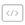• NumPy 基本操作实验 1 NumPy 数值计算基础入门

## 实验任务

• 了解 NumPy 数值类型与数据结构
• 使用 NumPy 对数组进行运算
• 使用 NumPy 对数组进行切片
• 使用 NumPy 对数组形状进行操作
• 使用 NumPy 对数组进行统计